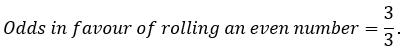It looks like you're using Internet Explorer 11 or older. This website works best with modern browsers such as the latest versions of Chrome, Firefox, Safari, and Edge. If you continue with this browser, you may see unexpected results.

# Math

## Probability

##### An event is defined as an outcome of an experiment. The collection of all outcomes of an experiment is called a sample space. The probability of an event is a number between 0 and 1. If the probability of an event is zero the event is called an impossible event. The probability of an event is a fraction given by:##### First, we write the sample space for this experiment:##### The number of two's in this sample space is one, therefore:##### The sample space is given by:##### there are three odd numbers in the sample space:. Therefore, the probability of rolling an odd number is:##### Two events A and A' are called complementary events if they satisfy the following equation:##### The sample space for this question is:. There is only one 3 in the sample space, therefore, the probability of rolling a 3 is:##### Complement of rolling a 3 is: not rolling a 3 with the following probability:##### Odds in favour of an event A is defined as:##### The even outcomes for this experiment are: 2, 4 and 6. Therefore, we get: